Next: Concluding remarks Up: Discussion and Conclusion Previous: Condensed matter and subthreshold

# Resonant molecular formation

Using the emitted beam of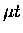from a hydrogen layer, we have measured the formation rates and resonance energy of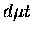muonic molecules. The combined results of the rate and energy scaling parameters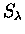, SE from two separate sets of runs are:

 = (122) SE = (123)

where the first errors are experimental uncertainties and the second ones are MC modeling uncertainties (including target geometry). When the errors are added in quadrature, we have achieved accuracies of about 25% and 10% respectively for the formation rates and energy. Our measurement of resonant molecular formation corresponds to a peak rateof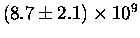s-1 for the reaction

 (124)

quantitatively confirming for the first time the existence of the strong epithermal resonance.

Our measurement of the resonance energy scaling corresponds to the position for the strongest the resonance peak in the reaction (9.6) of meV in thelab frame. If we assume the molecular spectrum of the complex [] is predicted reliably, our results can be considered a first direct measurement of the loosely bound state energy level. Our accuracy of meV in the center of mass frame, compared to the muonic atomic energy scale  of = = eV, is better than 10 ppm. Indeed it is comparable to the vacuum polarization correction in the loosely bound state energy level.

Until a few years ago, the problem of muonic molecule binding energies appeared to have been completely settled at least for the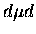case. Extraction of the binding energy from the temperature dependence offormation rates, as analyzed by Scrinzi et al. , showed remarkable agreement with the theoretical prediction. However, new studies, both theoretical and experimental, seem to indicate the real situation is not as clear. For example, recent calculations by Harston et al. on thefinite size effects indicates that previous values used in Ref.  were significantly underestimated. Similar effects are suggested for thecase by Bakalov et al. . Experimentally, new and precise measurements offusion at PSI  are not in complete agreement with those used in Ref. . In addition, the assumption of complete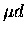thermalization may not be valid at low temperatures. It should also be stressed that in thecase, because of the lack of experimental observation of the temperature dependence predicted by the standard Vesman theory, there was virtually no experimental information on theenergy level. Thus our measurements of the resonance energy may provide an interesting new opportunity to test the calculations ofbinding energies.

Let us make a few remarks on the theoretical assumptions in our analysis.

• Inclusion of explicit back decay in the simulation is essential. The use of the renormalized effective rates without an explicit resonant scattering channel would lead to a significant overestimate in the calculated fusion yield. See appendix B for a detailed discussion.

• In our Monte Carlo simulations, the dependence of the formation rates and fusion probability W on the quantum states S, Kf are separately averaged, i.e., the averages for and W over the quantum states are factorized. This is not rigorously accurate if the their dependence on S or Kf is large. Although this is probably not a large effect, the precise estimate of the degree of accuracy requires comparisons with more complete calculations.

• Since our D2 target is not in thermal equilibrium rotationally (i.e., ) the use of Eq. 2.40-2.42 assuming the Boltzmann distribution for Kf may introduce some error, though judging from the difference in the results between using Eq. 2.40-2.42 and Eq. 2.37-2.39 at 300 K, shown in Figs. 10 and 11 of Ref. , the effects appear rather small. The Kfdistribution could also be affected if the rotational relaxation is substantially smaller than predicted by Ostrovskii and Ustimov , as suggested in Ref. .

• The use of a (state independent) effective fusion rate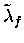appears valid at least for its Auger decay contribution, since it is presumably independent of , or S. However the value used, s-1, could be overestimated by %, if the lowest value amongst Armour's several predictions  turns out to be the correct one. This would not affect low energy formation where the fusion probability is very high, but could have a significant effect in epithermal formation, where fusion and back decay branches are comparable. On the other hand, possible collisional vibrational quenching would increase.

• Our method of scaling the formation rate, given in Eq. 8.8, while keeping the fusion probability W fixed, is phenomenological in nature. More physically motivated scaling would be to scale the matrix elements, which change both and W. By the same token, scaling of thebinding energy as input into the formation rate calculations, and comparisons with the resulting resonance structures (assuming scaled binding energies) may be more justified than our simple scaling in Eq. 8.9 for the resonance energy measurements, although these comparisons would require considerable input from theorists. Thus, our scaling results should be taken as a first step toward a more complete analysis.

• In our analysis, the resonance width is given by Faifman et al., which is determined by the Doppler broadening due to the D2 motion assuming a 3 K gas. In a solid, however, the Doppler broadening is expected to be larger due in part to the larger zero-point motion of a D2 bound in the lattice, hence the resonance profile may be broader. The precise evaluation of this effect would require more theoretical work which is in progress .

Finally, let us present in Fig. 9.3 a comparison of our data with the MC calculations assuming a constant rate forformation, a value of which was chosen to reproduce the fusion yield in the US moderator layer. The indication is quite convincing; we have confirmed the existence of epithermal resonantformation incollisions with D2.Next: Concluding remarks Up: Discussion and Conclusion Previous: Condensed matter and subthreshold